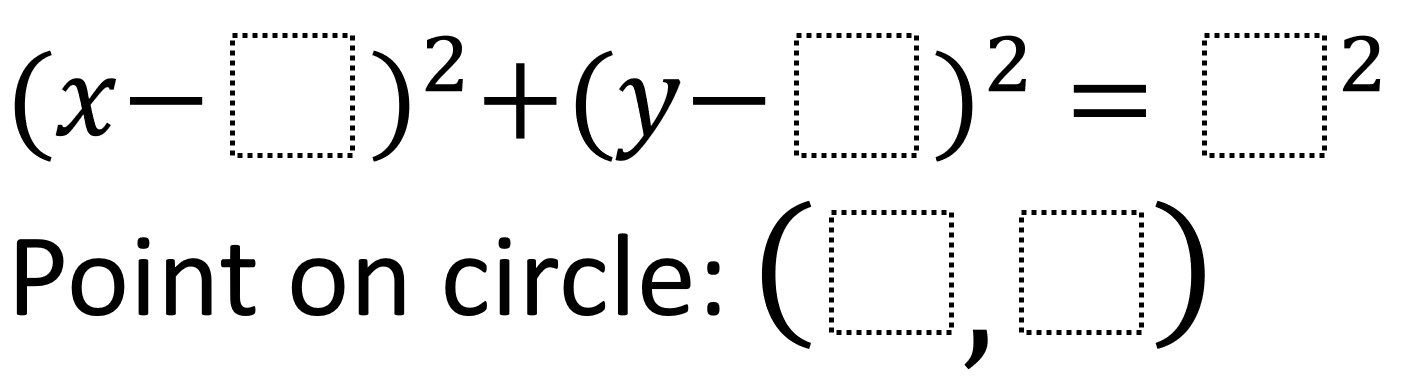# Equations of Circles 2

Directions: Using the integers -9 to 9, at most one time each, fill in the boxes to create a circle and a point on the circle with the point being as close to the origin as possible.### Hint

How can you use the values being subtracted from x and y to adjust where the circle is?